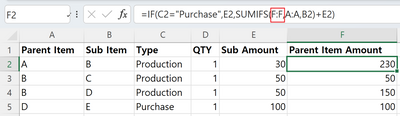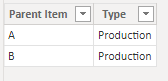cancel
Showing results for
Did you mean:Frequent Visitor

## BOM Calculation (Circular reference error)

Hi

This is a circular referencing Excel example.
This is a sample of costing by rolling up the BOM.
The purchased sub-item E goes into D, and D and C go into B.
Finally, B goes into A.
At this time, the same column F is referenced, but a circular reference error occurs in POWER BI.

No error occurs in Excel.

(check the excel formula)
I want to implement this in Power BI.
How can I do it?1 ACCEPTED SOLUTIONCommunity Support

Hi, @HJK

You can try the following methods.
New table:

``Table 2 = FILTER(SUMMARIZE('Table','Table'[Parent Item],'Table'[Type]),[Type]<>"Purchase")``Column:

``````Column =
Var _N1=CALCULATE(SUM('Table'[Sub Amount]),FILTER('Table',[Parent Item]=EARLIER('Table'[Sub Item])))
Var _N2=CALCULATE(SUM('Table'[Sub Amount]),ALLEXCEPT('Table','Table'[Parent Item],'Table'[Sub Item]))
Return
IF([Type]="Purchase",_N2,IF([Sub Item] in VALUES('Table 2'[Parent Item]),_N1+_N2+_N1,_N1+_N2))``````Is this the result you expect?

Best Regards,

Community Support Team _Charlotte

If this post helps, then please consider Accept it as the solution to help the other members find it more quickly.Community Support

Hi, @HJK

You can try the following methods.
New table:

``Table 2 = FILTER(SUMMARIZE('Table','Table'[Parent Item],'Table'[Type]),[Type]<>"Purchase")``Column:

``````Column =
Var _N1=CALCULATE(SUM('Table'[Sub Amount]),FILTER('Table',[Parent Item]=EARLIER('Table'[Sub Item])))
Var _N2=CALCULATE(SUM('Table'[Sub Amount]),ALLEXCEPT('Table','Table'[Parent Item],'Table'[Sub Item]))
Return
IF([Type]="Purchase",_N2,IF([Sub Item] in VALUES('Table 2'[Parent Item]),_N1+_N2+_N1,_N1+_N2))``````Is this the result you expect?

Best Regards,

Community Support Team _Charlotte

If this post helps, then please consider Accept it as the solution to help the other members find it more quickly.